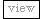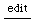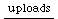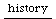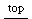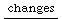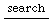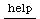## Code optimisation tips

Some generic tips on optimising / optimizing your SC code for efficient running:

### Lazy evaluation inside "if"

You want to use "if" to choose between possibilities - make sure you wrap the possibilities in curly brackets, so that they're functions and will therefore only be evaluated if they're needed:

```bench{1000000.do {a = if([true,false].choose, 10.0.rand.squared, 20.0.rand.squared)}}
bench{1000000.do {a = if([true,false].choose, {10.0.rand.squared}, {20.0.rand.squared})}}
```

### Replace && and || with and:{} and or:{}

Many languages use && and || as "short-circuit" operators, only evaluating the right-hand side if needed, but in SC the short-circuit only happens if you use and:{} or or:{} to pass the right-hand side as a function.

```{var a= 2; 100000.do{if(a==2 && a==2, {2}, {3})}}.bench
{var a= 2; 100000.do{if(a==2 and:{a==2}, {2}, {3})}}.bench
{var a= 2; 100000.do{if(a!=2 || a==2 && a==2, {2}, {3})}}.bench
{var a= 2; 100000.do{if(a!=2 or:{a==2 and:{a==2}}, {2}, {3})}}.bench
```

And of course, if the order doesn't matter, try to put the easy decisions at the left (e.g. a simple equality test) to make it less likely that the harder decisions need to be made explicitly.

### a * a is a little bit faster than a.squared

Note: it also behaves differently. SC, with audio signals in mind, returns a negative value when you apply .squared to a negative value!

```{var a= 2; 100000.do{a.squared}}.bench
{var a= 2; 100000.do{a*a}}.bench
```

### .sum takes a function (so does .mean)

Rather than iterating over an array and then summing the result, call .sum with the iteration to be used.

```({1000.do{([5.5, 3.7, 0.1]-[0, 1, 2]).abs.squared.sum}}.bench)
({1000.do{([5.5, 3.7, 0.1]-[0, 1, 2]).sum{|x| x.abs.squared}}}.bench)
```

### Avoid using argument keywords

Calling functions/methods is slightly faster if you pass the arguments in in their correct order, rather than using keywords.

```{100000.do{Buffer.new(server: s, bufnum: 3, numFrames: 100, numChannels: 2)}}.bench;
{100000.do{Buffer.new(s, 100, 2, 3)}}.bench;
{100000.do{Buffer.new(server: s, numFrames: 100, numChannels: 2, bufnum: 3)}}.bench;
```

Note: the speed difference is very, very small! In general you don't need to worry about this.

### all .isNil.not could be .notnil

```{var a= 2; 100000.do{if(a.isNil.not, {2}, {3})}}.bench
{var a= 2; 100000.do{if(a.notNil, {2}, {3})}}.bench
```

### {1 == ~var} seems to be slower than {~var == 1}

Not sure why.

```~boo = 15;
bench{1000000.do{~boo == 1}}
bench{1000000.do{1 == ~boo}}
```

### checking the presence of an item in an array

```r= [2, 3, 4]
{100000.do{r.includes(3)}}.bench
{100000.do{r.indexOf(3).notNil}}.bench	//faster
```

### set bits in a byte

```{1000000.do{100|1}}.bench				//fastest
{1000000.do{100.setBit(0)}}.bench
{1000000.do{100|128}}.bench				//fastest
{1000000.do{100.setBit(7)}}.bench
```

### replace pow(2), pow(3) and pow(4) if possible

```{var a= 2; 400000.do{a.squared}}.bench	//fast
{var a= 2; 400000.do{a.pow(2)}}.bench
{var a= 2; 400000.do{a*a}}.bench			//also as fast

{var a= 2; 400000.do{a.cubed}}.bench		//fastest!
{var a= 2; 400000.do{a.pow(3)}}.bench
{var a= 2; 400000.do{a*a*a}}.bench		//also fast

{var a= 2; 200000.do{a.pow(4)}}.bench
{var a= 2; 200000.do{a*a*a*a}}.bench		//fastest
```

But remember - in SC .squared returns a negative number if a negative is passed in, so a.squared is not necessarily the same as a.pow(2), but (a*a) is the same as a.pow(2).

```({var x;
x = [1,2,3,4,5,6,7,8,9,10];
100000.do({
x.sum;
})
}.bench; // ~ 0.26s
)

({var x1,x2,x3,x4,x5,x6,x7,x8,x9,x10;
#x1,x2,x3,x4,x5,x6,x7,x8,x9,x10 = [1,2,3,4,5,6,7,8,9,10];
100000.do({
x1+x2+x3+x4+x5+x6+x7+x8+x9+x10;
})
}.bench; // ~ 0.021s
)
```